吴恩达机器学习线性回归练习题：多变量线性回归（octave实现）

• 特征标准化，对应featureNormalize函数
• 作用：消除不同特征间的量纲，提高梯度下降的速度，缩短收敛时间
• 需要在featureNormalize.m文件中填充的代码：
mu = mean(X);    % 计算每个特征的均值
sigma = std(X);    % 计算每个特征的标准差
X_norm = (X - mu) ./ sigma;    % 计算标准化后的特征矩阵
• 多变量损失函数，对应computeCostMulti函数
• 作用：评估误差
• 损失函数定义：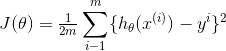，其中：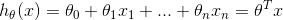• 这个公式可以有多种方式实现，这里根据习题中的介绍，使用矩阵计算
• 需要在computeCostMulti.m中填充的代码：
J = (X * theta - y)' * (X * theta - y) / (2 * m);    % 在进行矩阵计算的过程中，很自然的就实现了求和，也可以使用其他方式实现，比如：J = sum(power(X * theta - y, 2)) / (2 * m);
• 梯度下降
• 作用：最小化损失函数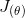，得到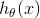中的所有参数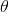，从而求得最佳拟合函数
•更新规则：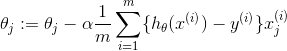• 梯度下降中迭代更新的实现方式有很多，这里采用矩阵计算的方式，一次计算，更新所有的theta = theta - ((X * theta -y)' * X)' * (alpha / m);

• 正规方程
• 作用：不通过梯度下降的方式计算出中的所有参数，从而求得最佳拟合函数
• 实现公式：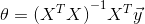• 根据公式直接实现即可
• 需要在normalEqn.m中填充的代码：
theta = inv(X' * X) * X' * y;    % 如果X' * X是奇异的，则需要使用pinv函数计算伪逆

• 在通过梯度下降方式计算出所有的后，要填充一下代码进行1650 sq-ft, 3 br 房屋的价格预测：
x_pre = [1650 3];    % 构建样本
x_pre_norm = (x_pre - mu) ./ sigma;    % 标准化数据
x_pre_norm = [ones(size(x_pre, 1)), x_pre_norm];    % 添加一个为1的特征

price = x_pre_norm * theta;    % 预测房屋价格

• 在通过正规方程计算出所有的后，要填充一下代码进行1650 sq-ft, 3 br 房屋的价格预测：
x_pre = [1 1650 3];
price = x_pre * theta;

Loading data ...
First 10 examples from the dataset:
x = [2104 3], y = 399900
x = [1600 3], y = 329900
x = [2400 3], y = 369000
x = [1416 2], y = 232000
x = [3000 4], y = 539900
x = [1985 4], y = 299900
x = [1534 3], y = 314900
x = [1427 3], y = 198999
x = [1380 3], y = 212000
x = [1494 3], y = 242500
Program paused. Press enter to continue.
Normalizing Features ...
334302.063993
100087.116006
3673.548451

Predicted price of a 1650 sq-ft, 3 br house (using gradient descent):
$289314.620338 Program paused. Press enter to continue. Solving with normal equations... Theta computed from the normal equations: 89597.909543 139.210674 -8738.019112 Predicted price of a 1650 sq-ft, 3 br house (using normal equations):$293081.464335

Python实现代码